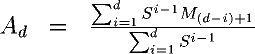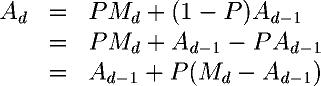Next: Calculating best fit Up: Calculating moving averages Previous: Weighted moving averages

### Exponentially smoothed moving averages

An exponentially smoothed moving average is a weighted moving average in which the weight factors are powers of S, the smoothing constant. An exponentially smoothed moving average is computed over all the data accumulated so far instead of being chopped off after some number of days. For day d the exponentially smoothed moving average is:But this is just a geometric sequence! The next term in such a sequence is given by: Ad=(1-S)Md+SAd-1. Calculation is expedited and comprehension served if we substitute: P=1-S for S into the equation for the next term. Doing a little algebra, we discover:This reformulation makes the operation of smoothing very intuitive. Every day, we take the old trend number Ad-1, calculate the difference between it and today's measurement Md, then add a percentage of that difference P to the old trend value obtain the new one. Obviously, the closer P is to 1 (and hence the closer S is to zero), the more influence the new measurement has upon the trend. If P=1, the old trend value Ad-1 cancels out and the moving average tracks the data precisely.

For example, with the smoothing constant S=0.9 we use on weight data, we calculate the new trend value Ad from the previous trend value Ad-1 and today's weight Md as:In discussions of exponentially smoothed moving averages, particularly their financial applications, beware of confusing the smoothing constant S with the variant form P=1-S introduced to simplify calculation and make the effect of the new data on the moving average more apparent. P is often referred to as the smoothing percentage''; the term 10% smoothing'' refers to a calculation in which P=10/100=0.1 and hence S=0.9.

By John Walker